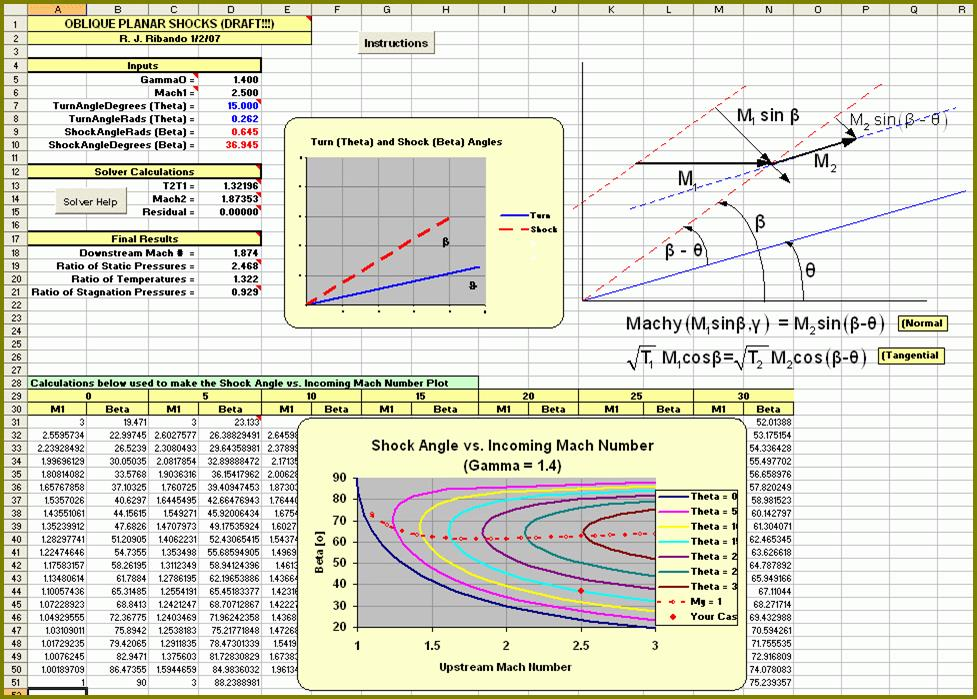# Oblique Planar Shocks

UPDATED: 08/29/2022

This Excel workbook will compute two-dimensional, oblique shocks. The user must be willing to learn Excel’s Solver Add-in in order to use it!  (All instructions are provided.) It is set up so that the user can easily input the upstream Mach number and turn angle (Θ). Using the Solver constraints, the user can solve for either the strong (M2 <1) or weak (M2 >1) solution.Interface for Excel/VBA solution for oblique shock waves. The geometry and nomenclature are shown in the upper right and the governing equations (normal and tangential directions) are seen below. The calculation for one set of conditions is at the top left. A plot of Shock Angle vs. Incoming Mach number for gamma = 1.4 is at the bottom